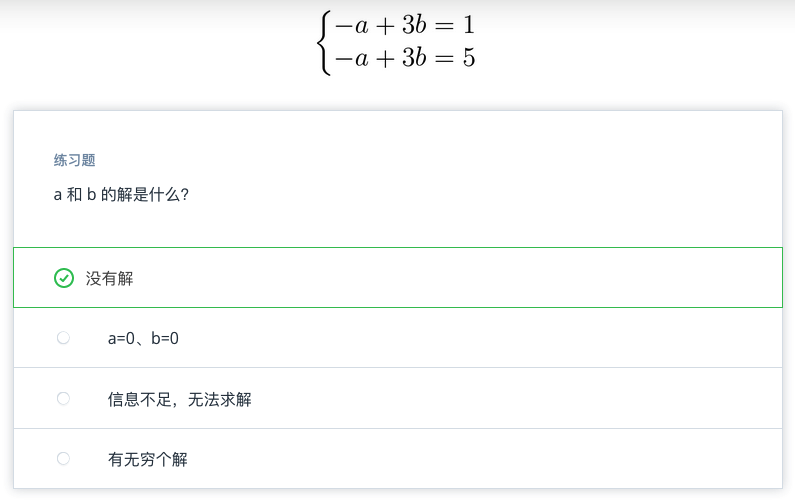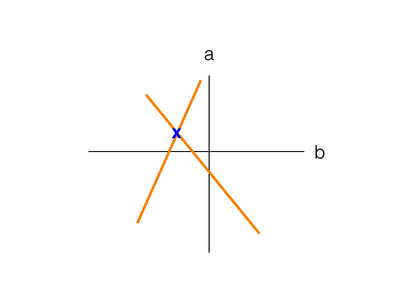# Python:线性代数-向量-求解简化的方程组 (四十六)

## 求解简化的方程组

• $\vec{x}=\begin{bmatrix} -14 \\2 \end{bmatrix}$

• $\vec{y}=\begin{bmatrix} 5 \\-1 \end{bmatrix}$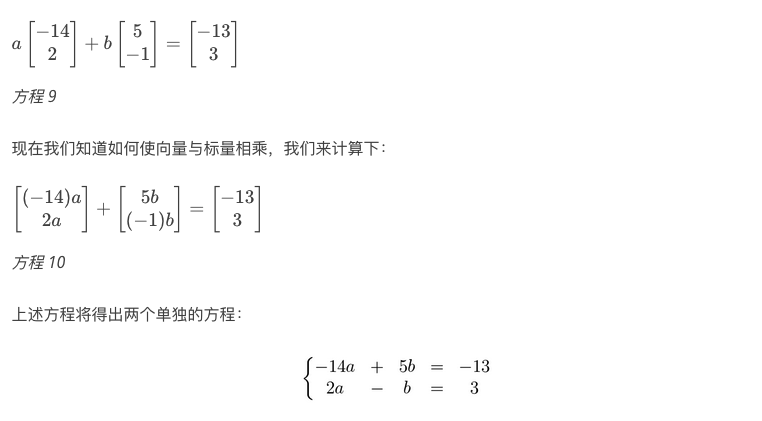- 图形法
- 替代法
- 消元法

## 图形法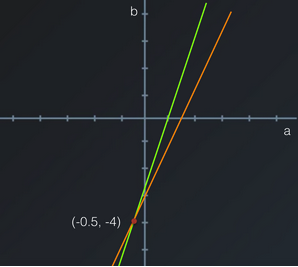a = −0.5 和 b = −4

## 替代法

(1) −14a + 5b = −13
(2) 2a − b = 3

−14a + 5(2a − 3) = −13 并求解 a。

## 消元法（注意：两个方程乘以的标量 bbb 即系数 bbb 的绝对值都是 5）。

−4a + 0b = 2

−4a = 2 ⇒ a = −0.5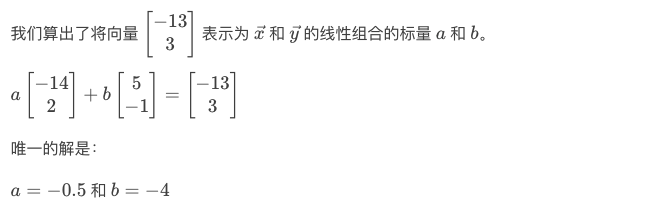## 练习题2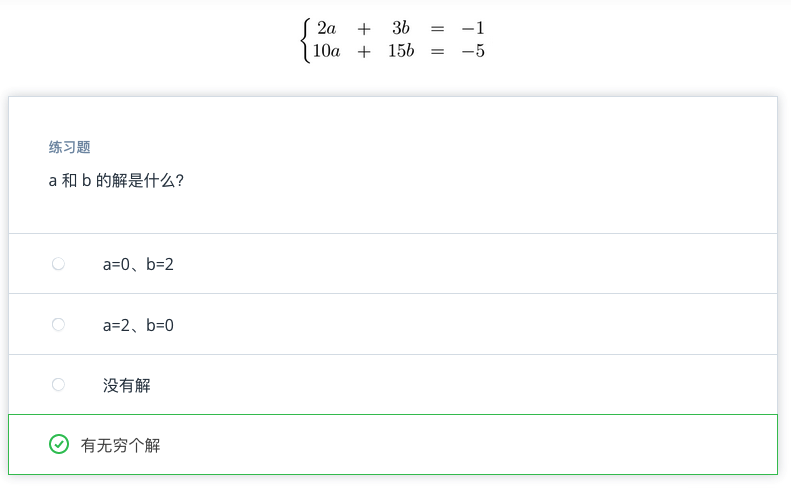## 练习题3